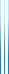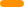# PublicationsPapers

## Low degree almost Boolean functions are sparse juntas

Irit Dinur, Yuval Filmus and Prahladh Harsha
Preliminary version in SODA'19

The Kindler–Safra theorem states that a constant degree almost Boolean function on $\{0,1\}^n$ is close to a junta. The theorem holds with respect to the $\mu_p$ measure whenever $p$ is bounded away from 0 and 1. When $p$ is very small, new phenomena emerge. For example, the sum of $\epsilon/p$ coordinates is $O(\epsilon^2)$-close to Boolean, yet is not close to a junta. My paper Friedgut–Kalai–Naor theorem for slices of the Boolean cube shows that this is essentially the only example in degree 1.

In this paper we generalize the Kindler–Safra theorem to the small $p$ regime. We show that a constant degree almost Boolean function is close to a constant degree sparse junta, a sparse polynomial having the property that on a random input, with high probability only a constant number of monomials are non-zero. As an application, we prove a large deviation bound, and show that constant degree Boolean functions are almost constant (though sparse juntas approximate them even better). Finally, we use our ideas to provide a new proof of the classical Kindler–Safra theorem.

A preliminary version of this work appeared in SODA’19. Unfortunately, that version relied on an agreement theorem whose proof contains a mistake (since corrected). The current version no longer relies on that agreement theorem. Instead, it employs a self-contained junta agreement theorem, following a suggestion of Dor Minzer.

## BibTeX

@inproceedings{DFH2019,
author = {Irit Dinur and Yuval Filmus and Prahladh Harsha},
title = {Analyzing boolean functions on the biased hypercube via higher-dimensional agreement tests},
booktitle = {ACM-SIAM Symposium on Discrete Algorithms (SODA19)},
year = {2019}
}

@misc{DFH2020+,
author = {Irit Dinur and Yuval Filmus and Prahladh Harsha},
title = {Low degree almost {B}oolean functions are sparse juntas},
year = {2020+}
}copy to clipboard